A Study on Steady Solutions of Nonlinear Wave

[How material is made from light]

[How did I get to 11 dimensional standing wave solution]

By recent experiments of high-energy accelerator it has already confirmed that ‘Material could be developed by collision of lights’.

From this matter we can guess that ‘All materials is made of light’, but it hasn’t confirmed.

I propose an idea here that ‘Material could be formed by the nonlinear electromagnetic wave as a phenomenon of self-organization’.

Of course ‘electromagnetic wave’ means lights.

It is known that the ‘speed of light in matter’ depends on the strength of the electromagnetic waves and it causes variety of non-linear effects.

Rectifier circuit is using nonlinearity of electronic devices.

I thoughts electromagnetic AC(alternating current)waves converts electromagnetic DC(direct current ) waves.

A kind of "rectification could be  happened  by it's nonlinearity in the matter.

Then I tried simulation such as the following.

Please browse 'nonlinear wave 1-dimensional animation'.

E_AC is AC electric field that has short wavelength.

E_DC is DC electric field that has long wavelength.

AC and DC electric fields are mixed.

AC electric field progresses from left to right.

DC electric field does not proceed. The initial value of the changing rate is 0.

The speed of light is normalizes to 1.

When the strength of electric field exceeds the constants E1, the speed of light decreases Δc(0.005).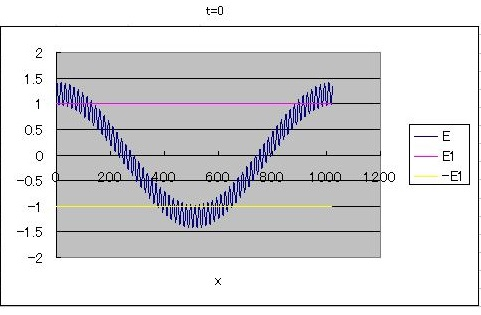According to Maxwell's equations I simulated using difference method.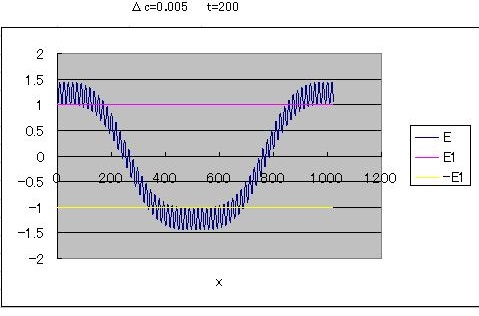"Rectification" is now maintained by the nonlinearity of DC electric field.

This is a phenomenon of self-organization.

Up to here the situation is in material.

But how about in vacuum space.

Generally, speed of light in a vacuum is considered constant.

But even if very strong electromagnetic fields, is speed of light really constant?

In the immediate near of proton or electron, electromagnetic field is very strong, who can measure the linearity in such situations?

I extended to a three-dimensional situation from Above-mentioned one-dimensional situation.

I seek E_DC Laplacian of Gaussian noise in the steady state.

Please see

E_DC Laplacian is derived as a function of E_DC,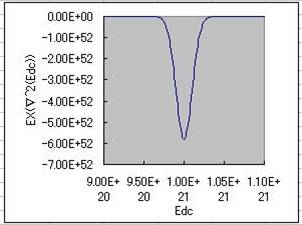Where the absolute value of E_DC is close to E1, the Laplacian of E_DC is large.

Its shape is same as a normal distribution.

With this formula, I found that the spherical solution that composed of radial electric field on the polar coordinates.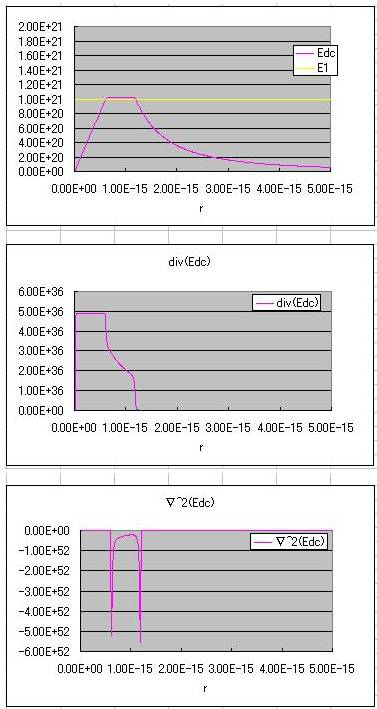I searched the K1 constant manually referring to the graph.

At large radius r, E( the electric field) is close to the asymptotic curves from a point charge.

At radius r is small, so that it reaches the E1 , and negative Laplacian E_DC.

Therefore E_DC bends. (Part of the right shoulder).

Then E_DC becomes nearly constant at slightly above E1.

When  E_DC decreases then Laplacian of E_DC increases.

This is a work of negative feedback.

Once again E_DC bend when radius r is smaller (the part of the left shoulder),

Then E_DC linearly proportional to the radius r.

I call the right shoulder to be "the outer shell",

and  I call the the left shoulder to be the inner shell"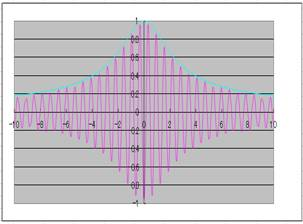A derivation of Dirac equation from standing waves  This solution is a combination of three 4 dimensional phenomena. And its amplitude is constant.

So the number of dimensions is 3 × 4 - 1 = 11.  In other words, it can be said to be an 11-dimensional phenomenon.